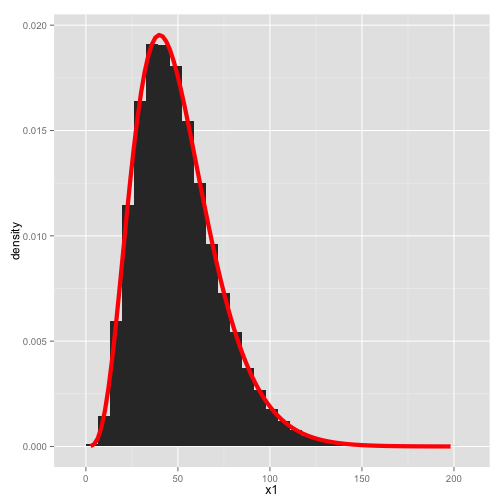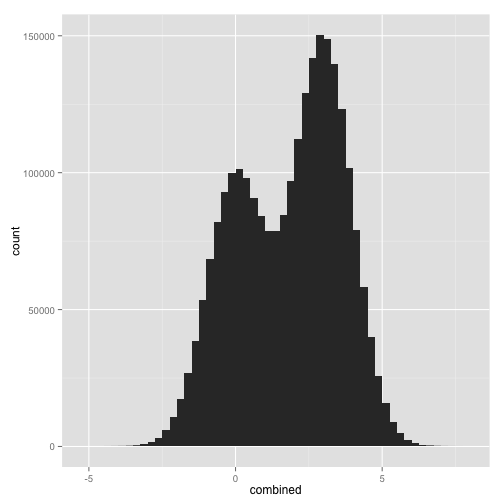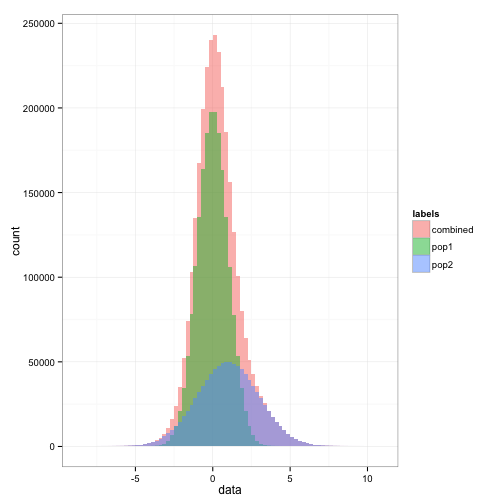# Lab 3: Simulations in R

In this lab, we'll learn how to simulate data with R using random number generators of different kinds of mixture variables we control.

## IMPORTANT

Unlike previous labs where the homework was done via OHMS, this lab will require you to submit short answers, submit plots (as aesthetic as possible!!), and also some code. All questions are numbered 1), 2), …

Formatting We prefer you try to make a markdown file with your answers (use MyName_Lab3.Rmd as your file) using Rstudio and the knitr function icon that will generate an html so that you can make aesthetic output easily. You then put these files (there will be an Rmd, a figure folder and an html to upload) in your dropbox folder on coursework.

## Getting started

When wanting to produce the same results with a random number generator it is important to set a starting point. This is important if you want to reproduce the results of a simulation or algorithm, and is very important in debugging.

Compare for instance the following output:

vecpoisson=rpois(100,5)
mean(vecpoisson)

##  4.74


If you do this alot, you get many different values. In fact if you put all the values together you would get what we call the sampling distribution of the mean of 100 Poisson random variables.

0) Why don't you get the same answer every time?

Now try:

set.seed(198911)
vecpoisson=rpois(100,5)
mean(vecpoisson)

##  4.8

set.seed(198911)
vecpoisson=rpois(100,5)
mean(vecpoisson)

##  4.8


## Using the apply function

Recall from probability that the sum of exponentials gives a gamma distribution. In this section, we will confirm that by simulation and cover some helpful functions in R. In general, we want to avoid for loops in R since that is slower than working with functions such as apply().

We will generate 5 samples from an exponential with a rate parameter 0.1 and sum them together. This is sum(rexp(n=nexps, rate=rate)). The function replicate() allows us to do this many times with very little code. Here, we do this sum 10,000 times to get an idea of the distribution.

reps <- 50000
nexps <- 5
rate <- 0.1
set.seed(0)
system.time(
x1 <- replicate(reps, sum(rexp(n=nexps, rate=rate)))
) # replicate

##    user  system elapsed
##   0.304   0.003   0.307

head(x1)

##  38.01 42.64 85.13 58.21 16.67 81.43


To confirm that this is correct, we can make a histogram or a qqplot of the simulation and compare it to what we know is the truth.

require(ggplot2)
ggplot(data.frame(x1), aes(x1)) +
geom_histogram(aes(y=..density..)) +
stat_function(fun=function(x)dgamma(x, shape=nexps, scale=1/rate),
color="red", size=2)There are different ways to do this. Look at the help(replicate) and you can see that there are various functions sapply(), lapply(), and vapply(). These are related to the functions apply() and tapply().

Here is how you might do the same thing with sapply(). You can plot this using the same commands above.

set.seed(0)
system.time(x1 <- sapply(1:reps, function(i){sum(rexp(n=nexps, rate=rate))})) # simple apply

##    user  system elapsed
##   0.277   0.017   0.295

head(x1)

##  38.01 42.64 85.13 58.21 16.67 81.43

set.seed(0)
system.time(x1 <- lapply(1:reps, function(i){sum(rexp(n=nexps, rate=rate))})) # list apply

##    user  system elapsed
##   0.231   0.001   0.232

head(x1)

## []
##  38.01
##
## []
##  42.64
##
## []
##  85.13
##
## []
##  58.21
##
## []
##  16.67
##
## []
##  81.43


When we apply a very simple function (e.g., a sum), the fastest way is often to just make a matrix of all the simulations and then apply that function to the matrix appropriately. The functions rowSums() and colSums() are particularly efficient at this.

set.seed(0)
system.time(x1 <- apply(matrix(rexp(n=nexps*reps, rate=rate), nrow=nexps),2,sum)) # apply on a matrix

##    user  system elapsed
##   0.114   0.003   0.117

head(x1)

##  38.01 42.64 85.13 58.21 16.67 81.43

set.seed(0)
system.time(x1 <- colSums(matrix(rexp(n=nexps*reps, rate=rate), nrow=nexps))) # using colSums

##    user  system elapsed
##   0.018   0.001   0.018

head(x1)

##  38.01 42.64 85.13 58.21 16.67 81.43


If you have a multi-core processor, sometimes you can speed things up by taking advantage of parallelization. In the package parallel is the function cmlapply() which acts very similarly to lapply(). By default, this uses only a single processor. You can change mc.cores if you have more cores.

require(parallel)

set.seed(0)
system.time(x1 <- mclapply(1:reps, function(i){sum(rexp(n=nexps, rate=rate))})) # multi-cluster apply

##    user  system elapsed
##   0.385   0.095   0.250

head(x1)

## []
##  28.92
##
## []
##  67.89
##
## []
##  26.47
##
## []
##  37.11
##
## []
##  31.21
##
## []
##  24.9


### Questions for this section

Do not use a for loop for any of these questions. You must show your code in order to get credit.

Question 1

Let $$U_1, U_2, U_3$$ all come from a uniform(0,1) distribution. Let $$M = \max(U_1, U_2, U_3)$$. Estimate (to 3 significant digits) the probability $$\mathbb{P}(M > 0.75)$$.

Question 2

Let $$Z_{(n)}$$ be maximum of $$n$$ standard normal observations. Estimate what $$n$$ should be so that $$\mathbb{P}(Z_{(n)} > 4) = 0.25$$.

Question 3

Let $$X_1, \dots, X_n$$ be Poisson random variables with parameter $$\lambda = 0.5$$ and where $$n=21$$. Estimate the probability that the sample mean is greater than the sample median.

Question 4

Let $$U$$ come from a uniform(0,1) distribution and $$Z$$ come from a standard normal distribution. Let $$X = pZ + (1-p)U$$. Estimate $$p$$ when $$X$$ has a variance of 0.4.

## Generating normal random variables

We will warm up by generating some random normal variables. Generate 1000 samples from the $$N(0,1)$$ distribution:

samples = rnorm(1000, 0, 1)


Question 5 Check that these are from $$N(0,1)$$ using a quantile-quantile plot (Q-Q plot). Use the stat_qq() function in the ggplot2 package.

Now load data that has been sampled from a “mystery” distribution. You can download the RData file here.

load("mystery_samples.RData")

##  27 29 31 26 30 18


Question 6 Which distribution do you think the samples comes from? Hint: look at the histogram, and the displot() function from the vcd package might be useful. You might also want to check the mean and variances.

## Generating Random Mixtures of Normal Data

Take a sample of size 1,000,000 from a Normal with mean 0 and variance 1 and a sample of size 1,500,000 from a normal with mean 3 and variance 1. Then plot the histogram with a binwidth of 0.25:

require(ggplot2)
sampa=rnorm(1000000,0,1)
sampb=rnorm(1500000,3,1)
combined = c(sampa, sampb)
plt = ggplot(data.frame(combined), aes(x=combined)) + stat_bin(binwidth=0.25, position="identity")
pltQuestion 7

• a) How many modes are there? Is this surprising?
• b) Now plot the density using geom_density().
• c) How is density related to hist?

Try making 3,000,000 points from a mixture of two normal groups with the means separated by 1 and the variance of the second group twice the size of the first. The first group should be twice the size of the second.

pop1=rnorm(2000000)
pop2=rnorm(1000000, 1, 2)
combined = c(pop1, pop2)
plt= ggplot(data.frame(data=c(combined, pop1, pop2), labels=rep(c("combined", "pop1", "pop2"), c(3e6, 2e6, 1e6))), aes(x=data)) + stat_bin(aes(fill=labels), position="identity", binwidth=0.25, alpha=0.5) + theme_bw()
pltQuestion 8

• a) What does this histogram show?
• b) Plot the densities of pop1 and pop2 on the same axes.

## Generating Random DNA strings

We want to generate a random DNA string with an independent background multinomial of $$(p_A,p_C,p_G,p_T)=(0.2,0.3,0.2,0.3)$$. Load the Biostrings package.

require(Biostrings)


Question 9 Show how to do this using sample().

Now we want to generate according to a Markov Chain with the following transition matrix:

trans=matrix(c(0.2, 0.2, 0.19, 0.1, 0.27, 0.34, 0.31, 0.34, 0.39,
0.24, 0.35, 0.36, 0.14, 0.22, 0.15, 0.20),nrow=4)
dimnames = list(c("A", "C", "G", "T"), c("A", "C", "G", "T"))
trans

##      [,1] [,2] [,3] [,4]
## [1,] 0.20 0.27 0.39 0.14
## [2,] 0.20 0.34 0.24 0.22
## [3,] 0.19 0.31 0.35 0.15
## [4,] 0.10 0.34 0.36 0.20


Begin by generating a nucleotide from the stationary distribution $$(p_A,p_C,p_G,p_T)=(0.18,0.325,0.325,0.18)$$. From here, generate the subsequent letters according to the chain's transition matrix.

Question 10 Generate a string of size 50 from this. Because we will be indexing into the transition matrix, it will be convenient to generate the indices instead of the alphabets.

## Generate two multivariate normal clusters

Generate two clusters with 100,000 points each:

require(MASS)
Sigma=matrix(c(5,3,3,2),2,2)
ex1=mvrnorm(100000,rep(0,2),Sigma)
Sigma=matrix(c(9,-5,-1,5),2,2)
ex2=mvrnorm(n=100000, rep(3, 2), Sigma)


Question 11 Plot both clusters on the same axes, along with 2d contours of their densities. You will want to use geom_point() and geom_density2d().

## Monte carlo simulation

We show how to compute the probability of simple events using simulation.

Suppose we rolled two fair dice. What is the probability that their sum is at least 7? We will approach this by simulating many throws of two fair dice, and then computing the fraction of those trials whose sum is at least 7. It will be convenient to write a function that simulates the trials and returns TRUE if the sum is at least 7 (we call this an event), and FALSE otherwise.

isEvent = function(numDice, numSides, targetValue, numTrials){
apply(matrix(sample(1:numSides, numDice*numTrials, replace=TRUE), nrow=numDice), 2, sum) >= targetValue
}


Now that we have our function, we are ready to do the monte carlo. It is good practice to set the random seed for reproducibility and debugging.

set.seed(0)
#try 5 trials
outcomes = isEvent(2, 6, 7, 5)
mean(outcomes)

##  1


This is far from the theoretical answer of $$\frac{21}{36}=0.58333$$. Now try with 10,000 trials:

set.seed(0)
outcomes = isEvent(2, 6, 7, 10000)
mean(outcomes)

##  0.5843


Ah, much better. The computation required for each trial was trivial, but in general, monte carlo simulations can be quite expensive, so we show how to easily parallelize the above computation in R using the “parallel” package.

require(parallel)


There is quite a bit to this package; we'll illustrate how to use pvec() for the dice example. We'll modify isEvent() so that it's fit for use with the pvec() function.

isEventPar = function(numDice, numSides, targetValue, trialIndices){
sapply(1:length(trialIndices), function(x) sum(sample(1:numSides, numDice, replace=TRUE)) >= targetValue)
}


We call pvec() with:

set.seed(0)
outcomes = pvec(1:10000, function(x) isEventPar(2, 6, 7, x))
mean(outcomes)

##  0.5931


As expected, the result is the same, provided we set the same random seed.

Question 12 For a coin with probability $$p$$ of landing heads, the expected number of tosses before we get the first head is $$\frac{1}{p}$$. Verify this with simulation for $$p=0.5$$.

## Gamma mixture of poissons

Generate the means of the poisson distribution by sampling from a gamma distribution:

lambdas = rgamma(100, shape=2, scale=3)
samples = rep(0, 100)
for (i in 1:100)
samples[i] = rpois(1, lambdas[i])


Question 13 Using the goodfit() and rootogram() functions in the vcd package, what distribution do you think fits the gamma-poisson mixture best? (Hint: Beware of the Chisquare test when some counts are smaller than 5.)

## Power calculation

The power of a statistical test is the probability that the test rejects the null hypothesis if the alternative is true. There is rarely a closed form for the power, so we resort to simulation. An important question in many clinical trials is how many subjects (samples) do we need to achieve a certain amount of power?

Suppose we want to find out how many samples are needed to distinguish between the means of two normal distributions, $$N(1, 0.5)$$ and $$N(2, 0.5)$$ with a power of at least 0.8 at the 0.05 significance level.

We'll take $$n$$ samples from each population, and compute the statistic $$\frac{\bar{X}_1-\bar{X}_2}{\sqrt{(0.5^2+0.5^2)/n}}$$. Under the null hypothesis that the two means are the same, this statistic has a $$N(0, 1)$$ distribution, and the $$p$$-value is $$2P\left(N(0,1)\geq\left|\frac{\bar{X}_1-\bar{X}_2}{\sqrt{(0.5^2+0.5^2)/n}}\right|\right)$$.

compute_power = function(n, sigma, numTrials){
sampa = matrix(rnorm(n*numTrials, 1, sigma), ncol=numTrials)
sampb= matrix(rnorm(n*numTrials, 2, sigma), ncol=numTrials)
statistics = (apply(sampa, 2, mean) - apply(sampb, 2, mean))/sqrt(2*sigma^2/n)
return (mean(abs(statistics) >= qnorm(0.975)))
}


Let's try 3 and 4 samples:

set.seed(0)
compute_power(3, 0.5, 10000)

##  0.6818

compute_power(4, 0.5, 10000)

##  0.8091


So it looks like 4 samples will do it.

Question 14 Will it take fewer or more samples if the standard deviations were 5 instead of 0.5? Verify this with simulation.